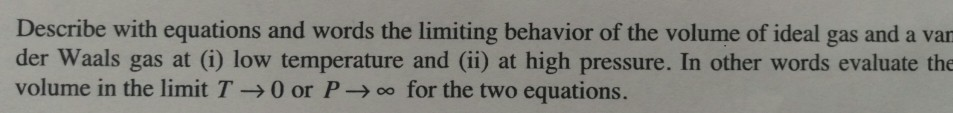# Question & Answer: Describe with equations and words the limiting behavior of the volume of ideal gas and a der…..Describe with equations and words the limiting behavior of the volume of ideal gas and a der Waals gas at (i) low temperature and (ii) at high pressure. In other words evaluate the volume in the limit T rightarrow 0 or P rightarrow infinity for the two equations.

for ideal gas PV = nRT

Don't use plagiarized sources. Get Your Custom Essay on
Question & Answer: Describe with equations and words the limiting behavior of the volume of ideal gas and a der…..
GET AN ESSAY WRITTEN FOR YOU FROM AS LOW AS \$13/PAGE

so as P –> infinity or T –> 0, volume tends to zero, as volume is directly propotional to temperature and inversly propotional to pressure.

for van der waal gas,

(P+an2/V2)*(V-nb) = nRT

as T–>0, V = nb

as P –> infinity, V = nb